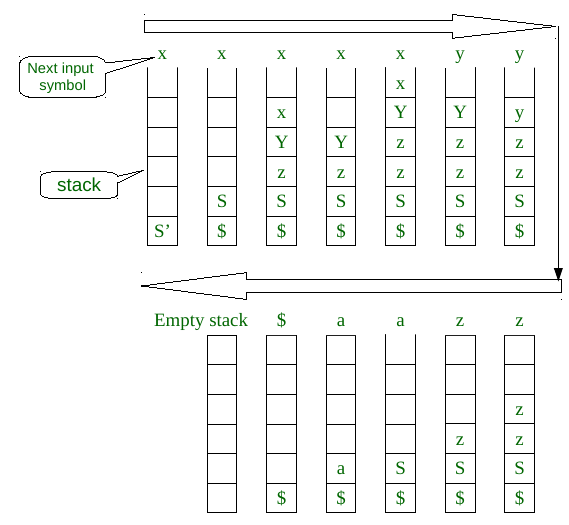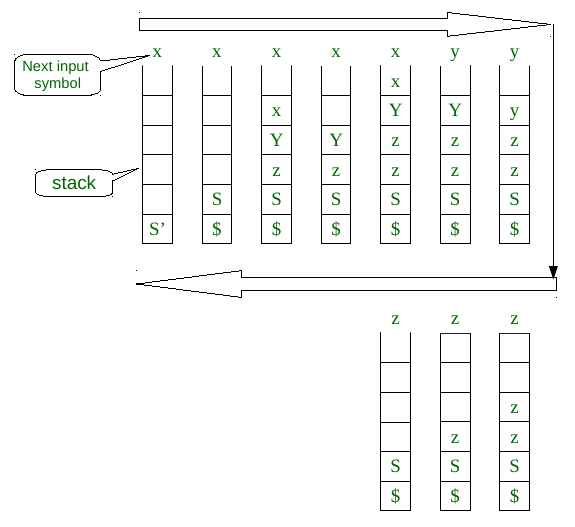Open in App
Not now

# LL(1) Parsing Algorithm

• Difficulty Level : Hard
• Last Updated : 29 Apr, 2021

Prerequisite — construction of LL(1) parsing table.
LL(1) parsing is a top-down parsing method in the syntax analysis phase of compiler design.  Required components for LL(1) parsing are input string, a stack, parsing table for given grammar, and parser. Here, we discuss a parser that determines that given string can be generated from a given grammar(or parsing table) or not.
Let given grammar is G = (V, T, S, P)
where V-variable symbol set, T-terminal symbol set, S- start symbol, P- production set.

LL(1) Parser algorithm:
Input- 1. stack = S  //stack initially contains only S.
2. input string = w\$
where S is the start symbol of grammar, w is given string, and \$ is used for the end of string.
3. PT is a parsing table of given grammar in the form of a matrix or 2D array.

Output- determines that given string can be produced by given grammar(parsing table) or not, if not then it produces an error.

Steps:

```1. while(stack is not empty) {

// initially it is S
2.     A = top symbol of stack;

//initially it is first symbol in string, it can be \$ also
3.     r = next input symbol of given string;
4.     if (A∈T or A==\$) {
5.         if(A==r){
6.             pop A from stack;
7.             remove r from input;
8.         }
9.         else
10.             ERROR();
11.     }
12.     else if (A∈V) {
13.         if(PT[A,r]= A⇢B1B2....Bk) {
14.             pop A from stack;

// B1 on top of stack at final of this step
15.             push Bk,Bk-1......B1 on stack
16.         }
17.         else if (PT[A,r] = error())
18.             error();
19.     }
20. }
// if parser terminate without error()
// then given string can generated by given parsing table.```

Time complexity
As we know that size of a grammar for a language is finite. Like, a finite number of variables, terminals, and productions. If grammar is finite then its LL(1) parsing table is also finite of size O(V*T).  Let

• p is the maximum of lengths of strings in RHS of all productions and
• l  is the length of given string and

l is very larger than p. if block at line 4 of algorithm always runs for O(1) time. else if block at line 12 in algorithm takes O(|P|*p) as upper bound for a single next input symbol. And while loop can run for more than l times, but we have considered the repeated while loop for a single next input symbol in O(|P|*p). So, the total time complexity is

```        T(l) = O(l)*O(|P|*p)
= O(l*|P|*p)
= O(l)           { as l >>>|P|*p }```

The time complexity of this algorithm is the order of length of the input string.

Comparison with Context-free language (CFL) :
Languages in LL(1) grammar is a proper subset of CFL. Using the CYK algorithm we can find membership of a string for a given Context-free grammar(CFG). CYK takes O(l3) time for the membership test for CFG. But for LL(1) grammar we can do a membership test in O(l) time which is linear using the above algorithm. If we know that given CFG is LL(1) grammar then use LL(1) parser for parsing rather than CYK algorithm.

Example –
Let the grammar G = (V, T, S’, P) is

```S' → S\$
S → xYzS | a
Y → xYz | y```

Parsing table(PT) for this grammar

`Let string1 = xxyzza,`

We have to add \$ with this string,
We will use the above parsing algorithm, diagram for the process :For string1 we got an empty stack, and while loop or algorithm terminated without error. So, string1 belongs to language for given grammar G.

`Let string2 = xxyzzz,`

Same way as above we will use the algorithm to parse the string2, here is the diagramFor string2, at the last stage as in the above diagram when the top of the stack is S and the next input symbol of the string is z, but in PT[S,z] = error. The algorithm terminated with an error. So, string2 is not in the language of grammar G

My Personal Notes arrow_drop_up
Related Articles